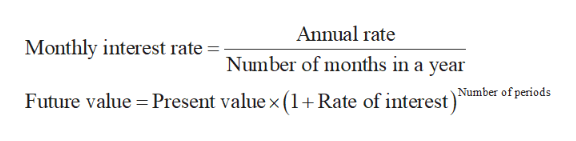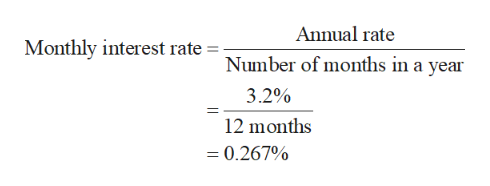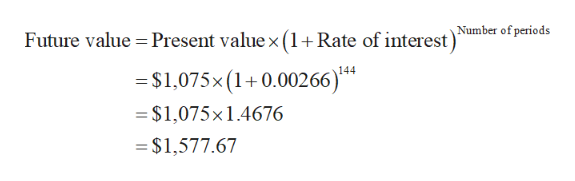# Lori buys a \$1,075 certificate of deposit (CD) that earns 3.2% interest that compounds monthly.  How much will the CD be worth in 12 years?

Question
2 views

Lori buys a \$1,075 certificate of deposit (CD) that earns 3.2% interest that compounds monthly.  How much will the CD be worth in 12 years?

check_circle

Step 1

Monthly interest rate and future value will be calculated using the formula,help_outlineImage TranscriptioncloseAnnual rate Monthly interest rate =- Number of months in a year Number of periods Future value Present value x (1 Rate of interest) fullscreen
Step 2

The monthly interest rate is calculated by dividing the annual rate with the number of months in a year. The annual rate of interest is 3.2% and hence, the monthly rate of interest comes out to be 0.267%help_outlineImage TranscriptioncloseAnnual rate Monthly interest rate = Number of months in a year 3.2% 12 months = 0.267% fullscreen
Step 3

The future value of a lump sum amount is the product of the present value and the future value compounding factor. The present value of ...help_outlineImage TranscriptioncloseNumber of periods Future value Present value x (1+ Rate of interest) \$1,075x (1+0.00266)*4 = \$1,075x1.4676 = \$1,577.67 fullscreen

### Want to see the full answer?

See Solution

#### Want to see this answer and more?

Solutions are written by subject experts who are available 24/7. Questions are typically answered within 1 hour.*

See Solution
*Response times may vary by subject and question.
Tagged in

### Math